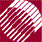ECE532 Biomedical Optics © 1998 Steven L. Jacques, Scott A. Prahl Oregon Graduate Institute

# E [W/cm2]

At a point of a surface, the radiant energy flux (or power) incident on an element of the surface, divided by the area of the surface.

The power P [W] that irradiates a surface area A [cm2] is called the Irradiance E [W/cm2]: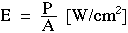# H [J/cm2]

At a point of a surface, the radiant energy incident on an element of the surface, divided by the area of the surface.

The energy Q [J] that reaches a surface area A [cm2] due to an irradiance E [W/cm2] maintained for a time duration t [s] is called the Radiant Exposure H [J/cm2]: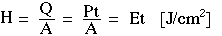The irradiance and radiant exposure are illustrated by the following figure. For a continuous source, a power P distributes over the target area A to yield irradiance E. For a pulsed source with pulse duration tp [s], a pulse energy Q distributes over the target area A to yield a radiant exposure H. The pulse energy Q is the integral of P(t) over time from 0 to tp. The pulse may be a perfect on/off rectangular pulse, or it may be a variable power P(t). Either way, Q is the total energy of the pulse.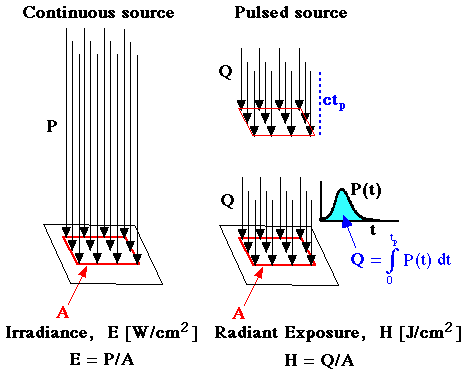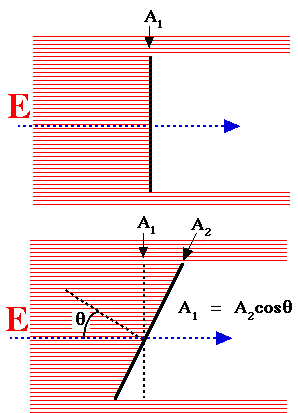The irradiance depends on the cross-sectional area which is perpendicular to the direction of irradiance. A surface which is oriented so there is an angle θ between the direction of irradiance and the normal to the surface will present a cross-sectional area Across-section = Acosθ. The power intercepted by the cross-sectional area of a target surface, Pintercepted, can be absorbed by that surface if it is absorbing or passed by that surface if it is an aperture. The irradiance equals:

E = Pintercepted/Across-section

In the figure, the power intercepted by the finite target surface areas A1 and A2 are the same because their cross-sectional areas are the same. The irradiance is the same at surfaces A1 and A2.

Often when discussing a light source, especially a collimated light source such as a laser or light from a distant source like the sun, the term irradiance is used to characterize the source. In this case, one is referring to the irradiance upon a virtual area (like A1 in this figure) which is oriented perpendicular to the direction of the source.

## Example:

Irradiance of a flashlight beam on a wall

There are several other radiometric parameters which are essentially the same as irradiance:

• Fluence rate